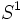# Knot invariant

This term is related to: knot theory

## Definition

A knot invariant is a function from the set of all knots (embeddings of$S^1$ in$S^3$) to some set, which is invariant under isotopy; in other words, if there is a self-homeomorphism of$S^3$, isotopic to the identity map, which sends one knot to the other, then the function should take the same value on both knots.

Note that isotopy-invariance is a weaker condition than being invariant under self-homeomorphisms, because the sphere possesses an orientation-reversing self-homeomorphism.

A list of knot invariants can be found at: